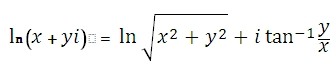# SQL Server IMLN function

Updated: 27 August 2010

Use IMLN to return the natural logarithm of a complex number in x + yi or x + yj text format. The natural logarithm of a complex number is computed as:Syntax
SELECT [wctEngineering].[wct].[IMLN] (
<@inumber, nvarchar(4000),>)
Arguments
@inumber
is the value at which to evaluate the function. @inumber is an expression of type varchar or of a type that can be implicitly converted to varchar and must be in the format x + yi or x + yj, where x is a real number that can be converted to float and yi (or yj) is an imaginary number, where the y part can be converted to float.
Return Types
varchar(4000)
Remarks
·         Use COMPLEX to convert real and imaginary coefficients into a complex number
Examples

select
wct.IMLN('7+24i')

This produces the following result
------------------------
3.2188758248682+1.28700221758657i

(1 row(s) affected)

### SupportCopyright 2008-2023 Westclintech LLC         Privacy Policy        Terms of Service